Publicité

# Part b1)Mass (kg)Velocity (ms)Force (N)Acceleration (ms2)Time to.docx

14 Nov 2022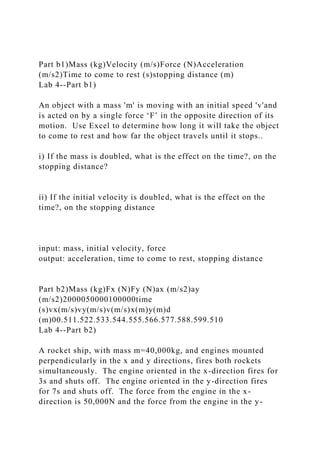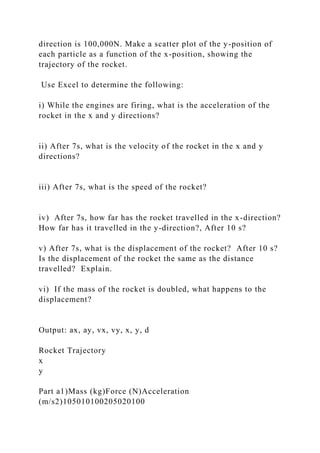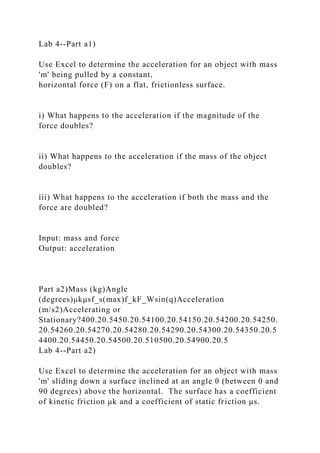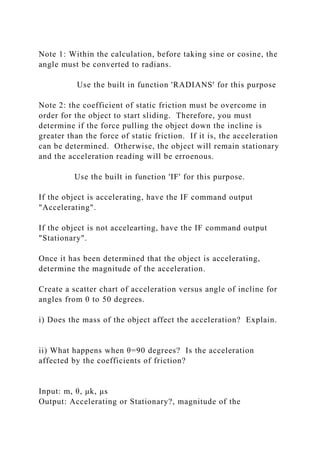Publicité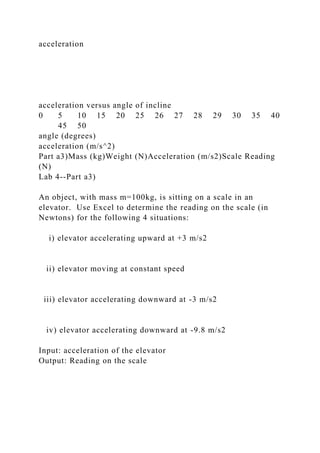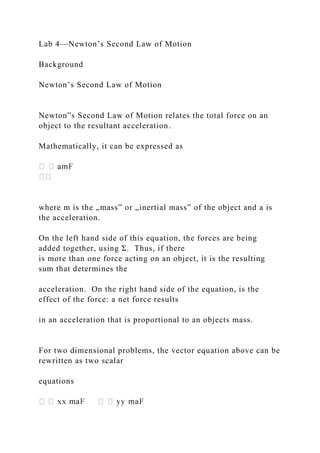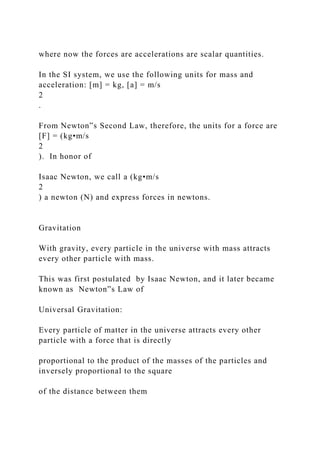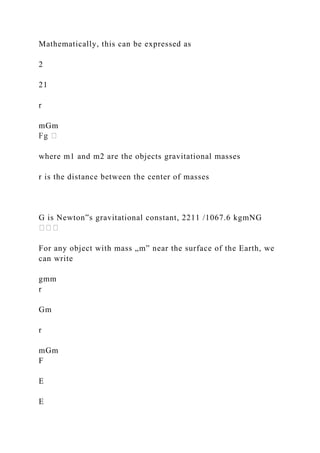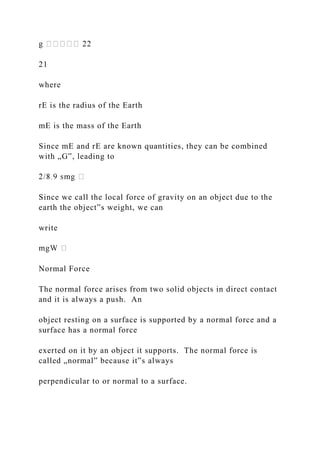Publicité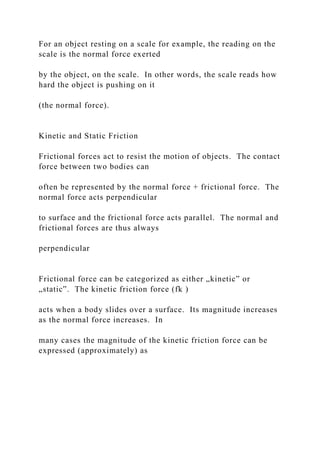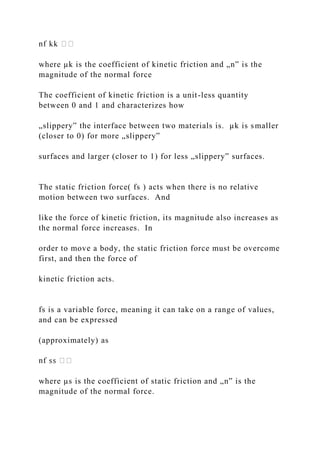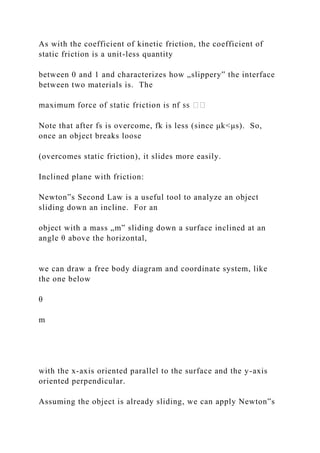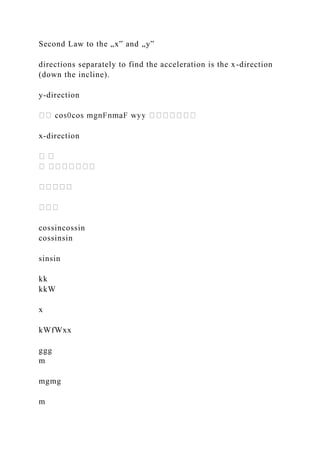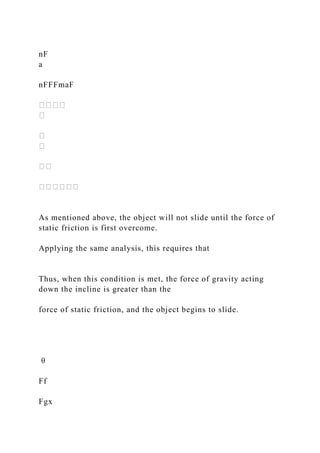Publicité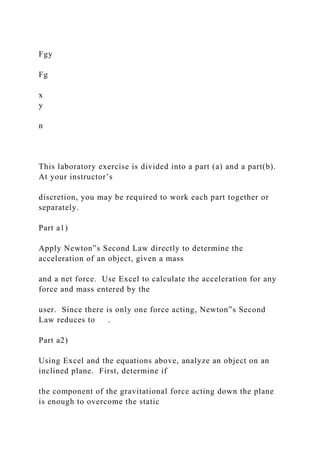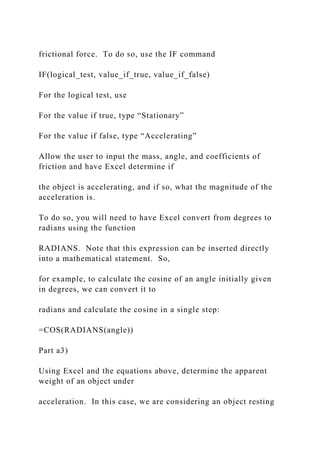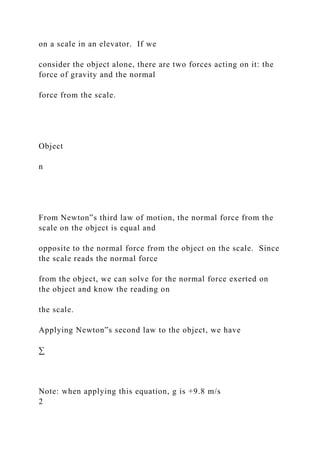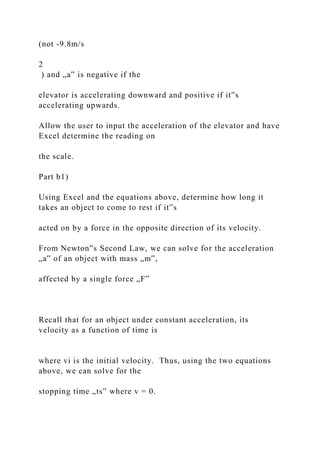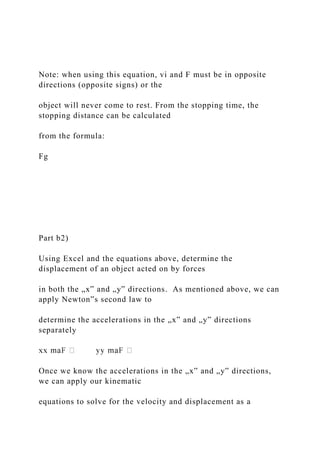Publicité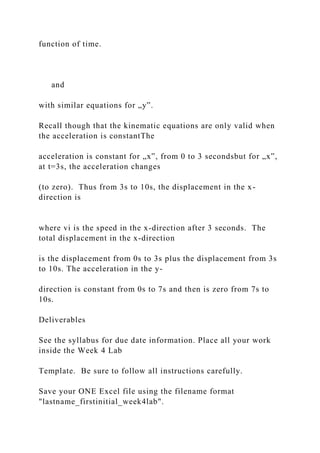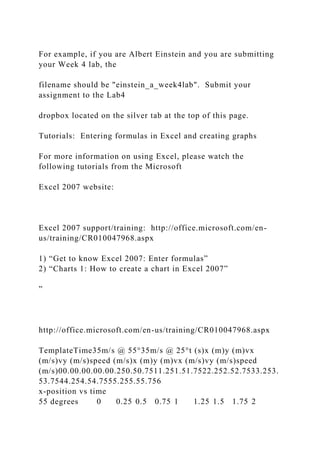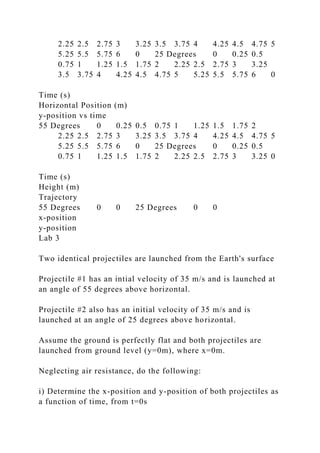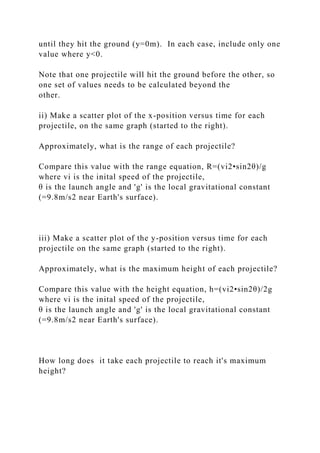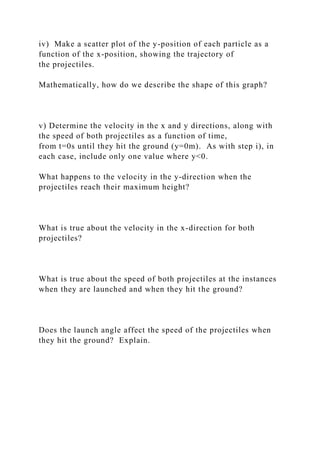Publicité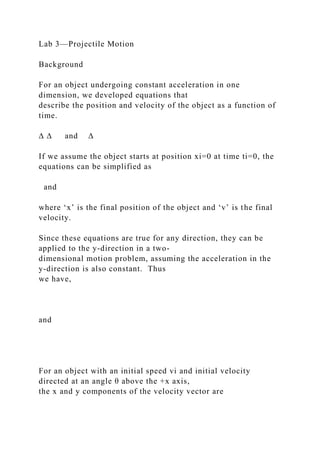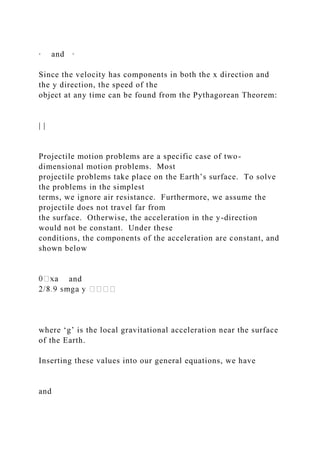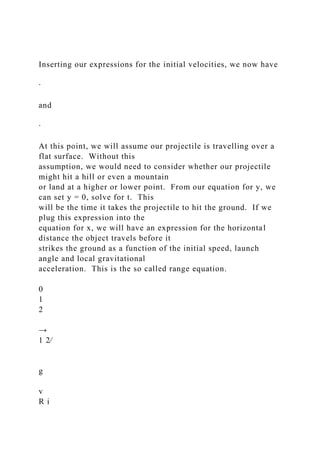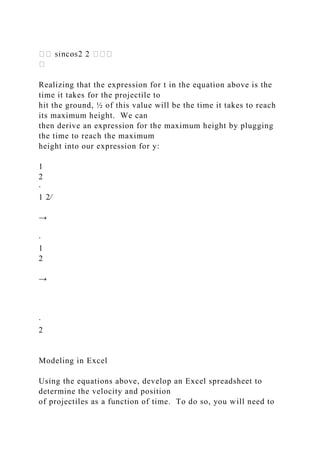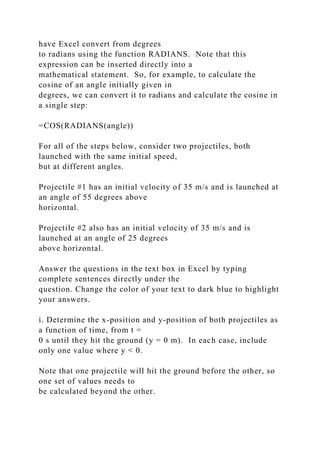Publicité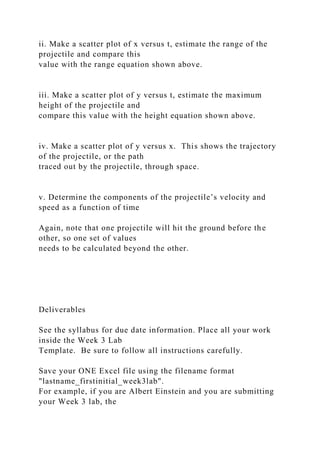Prochain SlideShare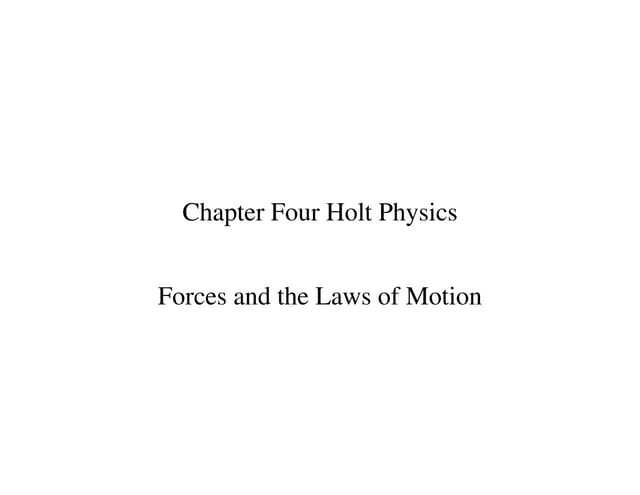Force_Lesson_Ch4.pdf
Chargement dans ... 3
1 sur 31
Publicité

### Part b1)Mass (kg)Velocity (ms)Force (N)Acceleration (ms2)Time to.docx

1. Part b1)Mass (kg)Velocity (m/s)Force (N)Acceleration (m/s2)Time to come to rest (s)stopping distance (m) Lab 4--Part b1) An object with a mass 'm' is moving with an initial speed 'v'and is acted on by a single force ‘F’ in the opposite direction of its motion. Use Excel to determine how long it will take the object to come to rest and how far the object travels until it stops.. i) If the mass is doubled, what is the effect on the time?, on the stopping distance? ii) If the initial velocity is doubled, what is the effect on the time?, on the stopping distance input: mass, initial velocity, force output: acceleration, time to come to rest, stopping distance Part b2)Mass (kg)Fx (N)Fy (N)ax (m/s2)ay (m/s2)2000050000100000time (s)vx(m/s)vy(m/s)v(m/s)x(m)y(m)d (m)00.511.522.533.544.555.566.577.588.599.510 Lab 4--Part b2) A rocket ship, with mass m=40,000kg, and engines mounted perpendicularly in the x and y directions, fires both rockets simultaneously. The engine oriented in the x-direction fires for 3s and shuts off. The engine oriented in the y-direction fires for 7s and shuts off. The force from the engine in the x- direction is 50,000N and the force from the engine in the y-
2. direction is 100,000N. Make a scatter plot of the y-position of each particle as a function of the x-position, showing the trajectory of the rocket. Use Excel to determine the following: i) While the engines are firing, what is the acceleration of the rocket in the x and y directions? ii) After 7s, what is the velocity of the rocket in the x and y directions? iii) After 7s, what is the speed of the rocket? iv) After 7s, how far has the rocket travelled in the x-direction? How far has it travelled in the y-direction?, After 10 s? v) After 7s, what is the displacement of the rocket? After 10 s? Is the displacement of the rocket the same as the distance travelled? Explain. vi) If the mass of the rocket is doubled, what happens to the displacement? Output: ax, ay, vx, vy, x, y, d Rocket Trajectory x y Part a1)Mass (kg)Force (N)Acceleration (m/s2)105010100205020100
3. Lab 4--Part a1) Use Excel to determine the acceleration for an object with mass 'm' being pulled by a constant, horizontal force (F) on a flat, frictionless surface. i) What happens to the acceleration if the magnitude of the force doubles? ii) What happens to the acceleration if the mass of the object doubles? iii) What happens to the acceleration if both the mass and the force are doubled? Input: mass and force Output: acceleration Part a2)Mass (kg)Angle (degrees)μkμsf_s(max)f_kF_Wsin(q)Acceleration (m/s2)Accelerating or Stationary?400.20.5450.20.54100.20.54150.20.54200.20.54250. 20.54260.20.54270.20.54280.20.54290.20.54300.20.54350.20.5 4400.20.54450.20.54500.20.510500.20.54900.20.5 Lab 4--Part a2) Use Excel to determine the acceleration for an object with mass 'm' sliding down a surface inclined at an angle θ (between 0 and 90 degrees) above the horizontal. The surface has a coefficient of kinetic friction μk and a coefficient of static friction μs.
4. Note 1: Within the calculation, before taking sine or cosine, the angle must be converted to radians. Use the built in function 'RADIANS' for this purpose Note 2: the coefficient of static friction must be overcome in order for the object to start sliding. Therefore, you must determine if the force pulling the object down the incline is greater than the force of static friction. If it is, the acceleration can be determined. Otherwise, the object will remain stationary and the acceleration reading will be erroenous. Use the built in function 'IF' for this purpose. If the object is accelerating, have the IF command output "Accelerating". If the object is not accelearting, have the IF command output "Stationary". Once it has been determined that the object is accelerating, determine the magnitude of the acceleration. Create a scatter chart of acceleration versus angle of incline for angles from 0 to 50 degrees. i) Does the mass of the object affect the acceleration? Explain. ii) What happens when θ=90 degrees? Is the acceleration affected by the coefficients of friction? Input: m, θ, μk, μs Output: Accelerating or Stationary?, magnitude of the
5. acceleration acceleration versus angle of incline 0 5 10 15 20 25 26 27 28 29 30 35 40 45 50 angle (degrees) acceleration (m/s^2) Part a3)Mass (kg)Weight (N)Acceleration (m/s2)Scale Reading (N) Lab 4--Part a3) An object, with mass m=100kg, is sitting on a scale in an elevator. Use Excel to determine the reading on the scale (in Newtons) for the following 4 situations: i) elevator accelerating upward at +3 m/s2 ii) elevator moving at constant speed iii) elevator accelerating downward at -3 m/s2 iv) elevator accelerating downward at -9.8 m/s2 Input: acceleration of the elevator Output: Reading on the scale
6. Lab 4—Newton’s Second Law of Motion Background Newton’s Second Law of Motion Newton‟s Second Law of Motion relates the total force on an object to the resultant acceleration. Mathematically, it can be expressed as where m is the „mass‟ or „inertial mass‟ of the object and a is the acceleration. On the left hand side of this equation, the forces are being added together, using Σ. Thus, if there is more than one force acting on an object, it is the resulting sum that determines the acceleration. On the right hand side of the equation, is the effect of the force: a net force results in an acceleration that is proportional to an objects mass. For two dimensional problems, the vector equation above can be rewritten as two scalar equations
7. where now the forces are accelerations are scalar quantities. In the SI system, we use the following units for mass and acceleration: [m] = kg, [a] = m/s 2 . From Newton‟s Second Law, therefore, the units for a force are [F] = (kg•m/s 2 ). In honor of Isaac Newton, we call a (kg•m/s 2 ) a newton (N) and express forces in newtons. Gravitation With gravity, every particle in the universe with mass attracts every other particle with mass. This was first postulated by Isaac Newton, and it later became known as Newton‟s Law of Universal Gravitation: Every particle of matter in the universe attracts every other particle with a force that is directly proportional to the product of the masses of the particles and inversely proportional to the square of the distance between them
8. Mathematically, this can be expressed as 2 21 r mGm where m1 and m2 are the objects gravitational masses r is the distance between the center of masses G is Newton‟s gravitational constant, 2211 /1067.6 kgmNG For any object with mass „m‟ near the surface of the Earth, we can write gmm r Gm r mGm F E E
9. 21 where rE is the radius of the Earth mE is the mass of the Earth Since mE and rE are known quantities, they can be combined with „G‟, leading to Since we call the local force of gravity on an object due to the earth the object‟s weight, we can write Normal Force The normal force arises from two solid objects in direct contact and it is always a push. An object resting on a surface is supported by a normal force and a surface has a normal force exerted on it by an object it supports. The normal force is called „normal‟ because it‟s always perpendicular to or normal to a surface.
10. For an object resting on a scale for example, the reading on the scale is the normal force exerted by the object, on the scale. In other words, the scale reads how hard the object is pushing on it (the normal force). Kinetic and Static Friction Frictional forces act to resist the motion of objects. The contact force between two bodies can often be represented by the normal force + frictional force. The normal force acts perpendicular to surface and the frictional force acts parallel. The normal and frictional forces are thus always perpendicular Frictional force can be categorized as either „kinetic‟ or „static‟. The kinetic friction force (fk ) acts when a body slides over a surface. Its magnitude increases as the normal force increases. In many cases the magnitude of the kinetic friction force can be expressed (approximately) as
11. where µk is the coefficient of kinetic friction and „n‟ is the magnitude of the normal force The coefficient of kinetic friction is a unit-less quantity between 0 and 1 and characterizes how „slippery‟ the interface between two materials is. µk is smaller (closer to 0) for more „slippery‟ surfaces and larger (closer to 1) for less „slippery‟ surfaces. The static friction force( fs ) acts when there is no relative motion between two surfaces. And like the force of kinetic friction, its magnitude also increases as the normal force increases. In order to move a body, the static friction force must be overcome first, and then the force of kinetic friction acts. fs is a variable force, meaning it can take on a range of values, and can be expressed (approximately) as where µs is the coefficient of static friction and „n‟ is the magnitude of the normal force.
12. As with the coefficient of kinetic friction, the coefficient of static friction is a unit-less quantity between 0 and 1 and characterizes how „slippery‟ the interface between two materials is. The Note that after fs is overcome, fk is less (since μk<μs). So, once an object breaks loose (overcomes static friction), it slides more easily. Inclined plane with friction: Newton‟s Second Law is a useful tool to analyze an object sliding down an incline. For an object with a mass „m‟ sliding down a surface inclined at an angle θ above the horizontal, we can draw a free body diagram and coordinate system, like the one below θ m with the x-axis oriented parallel to the surface and the y-axis oriented perpendicular. Assuming the object is already sliding, we can apply Newton‟s
13. Second Law to the „x‟ and „y‟ directions separately to find the acceleration is the x-direction (down the incline). y-direction x-direction cossincossin cossinsin sinsin kk kkW x kWfWxx ggg m mgmg m
14. nF a nFFFmaF As mentioned above, the object will not slide until the force of static friction is first overcome. Applying the same analysis, this requires that Thus, when this condition is met, the force of gravity acting down the incline is greater than the force of static friction, and the object begins to slide. θ Ff Fgx
15. Fgy Fg x y n This laboratory exercise is divided into a part (a) and a part(b). At your instructor’s discretion, you may be required to work each part together or separately. Part a1) Apply Newton‟s Second Law directly to determine the acceleration of an object, given a mass and a net force. Use Excel to calculate the acceleration for any force and mass entered by the user. Since there is only one force acting, Newton‟s Second Law reduces to . Part a2) Using Excel and the equations above, analyze an object on an inclined plane. First, determine if the component of the gravitational force acting down the plane is enough to overcome the static
16. frictional force. To do so, use the IF command IF(logical_test, value_if_true, value_if_false) For the logical test, use For the value if true, type “Stationary” For the value if false, type “Accelerating” Allow the user to input the mass, angle, and coefficients of friction and have Excel determine if the object is accelerating, and if so, what the magnitude of the acceleration is. To do so, you will need to have Excel convert from degrees to radians using the function RADIANS. Note that this expression can be inserted directly into a mathematical statement. So, for example, to calculate the cosine of an angle initially given in degrees, we can convert it to radians and calculate the cosine in a single step: =COS(RADIANS(angle)) Part a3) Using Excel and the equations above, determine the apparent weight of an object under acceleration. In this case, we are considering an object resting
17. on a scale in an elevator. If we consider the object alone, there are two forces acting on it: the force of gravity and the normal force from the scale. Object n From Newton‟s third law of motion, the normal force from the scale on the object is equal and opposite to the normal force from the object on the scale. Since the scale reads the normal force from the object, we can solve for the normal force exerted on the object and know the reading on the scale. Applying Newton‟s second law to the object, we have ∑ Note: when applying this equation, g is +9.8 m/s 2
18. (not -9.8m/s 2 ) and „a‟ is negative if the elevator is accelerating downward and positive if it‟s accelerating upwards. Allow the user to input the acceleration of the elevator and have Excel determine the reading on the scale. Part b1) Using Excel and the equations above, determine how long it takes an object to come to rest if it‟s acted on by a force in the opposite direction of its velocity. From Newton‟s Second Law, we can solve for the acceleration „a‟ of an object with mass „m‟, affected by a single force „F‟ Recall that for an object under constant acceleration, its velocity as a function of time is where vi is the initial velocity. Thus, using the two equations above, we can solve for the stopping time „ts‟ where v = 0.
19. Note: when using this equation, vi and F must be in opposite directions (opposite signs) or the object will never come to rest. From the stopping time, the stopping distance can be calculated from the formula: Fg Part b2) Using Excel and the equations above, determine the displacement of an object acted on by forces in both the „x‟ and „y‟ directions. As mentioned above, we can apply Newton‟s second law to determine the accelerations in the „x‟ and „y‟ directions separately Once we know the accelerations in the „x‟ and „y‟ directions, we can apply our kinematic equations to solve for the velocity and displacement as a
20. function of time. and with similar equations for „y‟. Recall though that the kinematic equations are only valid when the acceleration is constantThe acceleration is constant for „x‟, from 0 to 3 secondsbut for „x‟, at t=3s, the acceleration changes (to zero). Thus from 3s to 10s, the displacement in the x- direction is where vi is the speed in the x-direction after 3 seconds. The total displacement in the x-direction is the displacement from 0s to 3s plus the displacement from 3s to 10s. The acceleration in the y- direction is constant from 0s to 7s and then is zero from 7s to 10s. Deliverables See the syllabus for due date information. Place all your work inside the Week 4 Lab Template. Be sure to follow all instructions carefully. Save your ONE Excel file using the filename format "lastname_firstinitial_week4lab".
21. For example, if you are Albert Einstein and you are submitting your Week 4 lab, the filename should be "einstein_a_week4lab". Submit your assignment to the Lab4 dropbox located on the silver tab at the top of this page. Tutorials: Entering formulas in Excel and creating graphs For more information on using Excel, please watch the following tutorials from the Microsoft Excel 2007 website: Excel 2007 support/training: http://office.microsoft.com/en- us/training/CR010047968.aspx 1) “Get to know Excel 2007: Enter formulas” 2) “Charts 1: How to create a chart in Excel 2007” ” http://office.microsoft.com/en-us/training/CR010047968.aspx TemplateTime35m/s @ 55°35m/s @ 25°t (s)x (m)y (m)vx (m/s)vy (m/s)speed (m/s)x (m)y (m)vx (m/s)vy (m/s)speed (m/s)00.00.00.00.00.250.50.7511.251.51.7522.252.52.7533.253. 53.7544.254.54.7555.255.55.756 x-position vs time 55 degrees 0 0.25 0.5 0.75 1 1.25 1.5 1.75 2
22. 2.25 2.5 2.75 3 3.25 3.5 3.75 4 4.25 4.5 4.75 5 5.25 5.5 5.75 6 0 25 Degrees 0 0.25 0.5 0.75 1 1.25 1.5 1.75 2 2.25 2.5 2.75 3 3.25 3.5 3.75 4 4.25 4.5 4.75 5 5.25 5.5 5.75 6 0 Time (s) Horizontal Position (m) y-position vs time 55 Degrees 0 0.25 0.5 0.75 1 1.25 1.5 1.75 2 2.25 2.5 2.75 3 3.25 3.5 3.75 4 4.25 4.5 4.75 5 5.25 5.5 5.75 6 0 25 Degrees 0 0.25 0.5 0.75 1 1.25 1.5 1.75 2 2.25 2.5 2.75 3 3.25 0 Time (s) Height (m) Trajectory 55 Degrees 0 0 25 Degrees 0 0 x-position y-position Lab 3 Two identical projectiles are launched from the Earth's surface Projectile #1 has an intial velocity of 35 m/s and is launched at an angle of 55 degrees above horizontal. Projectile #2 also has an initial velocity of 35 m/s and is launched at an angle of 25 degrees above horizontal. Assume the ground is perfectly flat and both projectiles are launched from ground level (y=0m), where x=0m. Neglecting air resistance, do the following: i) Determine the x-position and y-position of both projectiles as a function of time, from t=0s
23. until they hit the ground (y=0m). In each case, include only one value where y<0. Note that one projectile will hit the ground before the other, so one set of values needs to be calculated beyond the other. ii) Make a scatter plot of the x-position versus time for each projectile, on the same graph (started to the right). Approximately, what is the range of each projectile? Compare this value with the range equation, R=(vi2•sin2θ)/g where vi is the inital speed of the projectile, θ is the launch angle and 'g' is the local gravitational constant (=9.8m/s2 near Earth's surface). iii) Make a scatter plot of the y-position versus time for each projectile on the same graph (started to the right). Approximately, what is the maximum height of each projectile? Compare this value with the height equation, h=(vi2•sin2θ)/2g where vi is the inital speed of the projectile, θ is the launch angle and 'g' is the local gravitational constant (=9.8m/s2 near Earth's surface). How long does it take each projectile to reach it's maximum height?
24. iv) Make a scatter plot of the y-position of each particle as a function of the x-position, showing the trajectory of the projectiles. Mathematically, how do we describe the shape of this graph? v) Determine the velocity in the x and y directions, along with the speed of both projectiles as a function of time, from t=0s until they hit the ground (y=0m). As with step i), in each case, include only one value where y<0. What happens to the velocity in the y-direction when the projectiles reach their maximum height? What is true about the velocity in the x-direction for both projectiles? What is true about the speed of both projectiles at the instances when they are launched and when they hit the ground? Does the launch angle affect the speed of the projectiles when they hit the ground? Explain.
25. Lab 3—Projectile Motion Background For an object undergoing constant acceleration in one dimension, we developed equations that describe the position and velocity of the object as a function of time. ∆ ∆ and ∆ If we assume the object starts at position xi=0 at time ti=0, the equations can be simplified as and where ‘x’ is the final position of the object and ‘v’ is the final velocity. Since these equations are true for any direction, they can be applied to the y-direction in a two- dimensional motion problem, assuming the acceleration in the y-direction is also constant. Thus we have, and For an object with an initial speed vi and initial velocity directed at an angle θ above the +x axis, the x and y components of the velocity vector are
26. ∙ and ∙ Since the velocity has components in both the x direction and the y direction, the speed of the object at any time can be found from the Pythagorean Theorem: | | Projectile motion problems are a specific case of two- dimensional motion problems. Most projectile problems take place on the Earth’s surface. To solve the problems in the simplest terms, we ignore air resistance. Furthermore, we assume the projectile does not travel far from the surface. Otherwise, the acceleration in the y-direction would not be constant. Under these conditions, the components of the acceleration are constant, and shown below and where ‘g’ is the local gravitational acceleration near the surface of the Earth. Inserting these values into our general equations, we have and
27. Inserting our expressions for the initial velocities, we now have ∙ and ∙ At this point, we will assume our projectile is travelling over a flat surface. Without this assumption, we would need to consider whether our projectile might hit a hill or even a mountain or land at a higher or lower point. From our equation for y, we can set y = 0, solve for t. This will be the time it takes the projectile to hit the ground. If we plug this expression into the equation for x, we will have an expression for the horizontal distance the object travels before it strikes the ground as a function of the initial speed, launch angle and local gravitational acceleration. This is the so called range equation. 0 1 2 → 1 2⁄ g v R i
28. Realizing that the expression for t in the equation above is the time it takes for the projectile to hit the ground, ½ of this value will be the time it takes to reach its maximum height. We can then derive an expression for the maximum height by plugging the time to reach the maximum height into our expression for y: 1 2 ∙ 1 2⁄ → ∙ 1 2 → ∙ 2 Modeling in Excel Using the equations above, develop an Excel spreadsheet to determine the velocity and position of projectiles as a function of time. To do so, you will need to
29. have Excel convert from degrees to radians using the function RADIANS. Note that this expression can be inserted directly into a mathematical statement. So, for example, to calculate the cosine of an angle initially given in degrees, we can convert it to radians and calculate the cosine in a single step: =COS(RADIANS(angle)) For all of the steps below, consider two projectiles, both launched with the same initial speed, but at different angles. Projectile #1 has an initial velocity of 35 m/s and is launched at an angle of 55 degrees above horizontal. Projectile #2 also has an initial velocity of 35 m/s and is launched at an angle of 25 degrees above horizontal. Answer the questions in the text box in Excel by typing complete sentences directly under the question. Change the color of your text to dark blue to highlight your answers. i. Determine the x-position and y-position of both projectiles as a function of time, from t = 0 s until they hit the ground (y = 0 m). In each case, include only one value where y < 0. Note that one projectile will hit the ground before the other, so one set of values needs to be calculated beyond the other.
30. ii. Make a scatter plot of x versus t, estimate the range of the projectile and compare this value with the range equation shown above. iii. Make a scatter plot of y versus t, estimate the maximum height of the projectile and compare this value with the height equation shown above. iv. Make a scatter plot of y versus x. This shows the trajectory of the projectile, or the path traced out by the projectile, through space. v. Determine the components of the projectile’s velocity and speed as a function of time Again, note that one projectile will hit the ground before the other, so one set of values needs to be calculated beyond the other. Deliverables See the syllabus for due date information. Place all your work inside the Week 3 Lab Template. Be sure to follow all instructions carefully. Save your ONE Excel file using the filename format "lastname_firstinitial_week3lab". For example, if you are Albert Einstein and you are submitting your Week 3 lab, the
31. filename should be "einstein_a_week3lab". Submit your assignment to the Lab3 dropbox located on the silver tab at the top of this page. Tutorials: Entering formulas in Excel and creating graphs For more information on using Excel, please watch the following tutorials from the Microsoft Excel 2007 website: Excel 2007 support/training: http://office.microsoft.com/en- us/training/CR010047968.aspx 1) “Get to know Excel 2007: Enter formulas” 2) “Charts 1: How to create a chart in Excel 2007”
Publicité